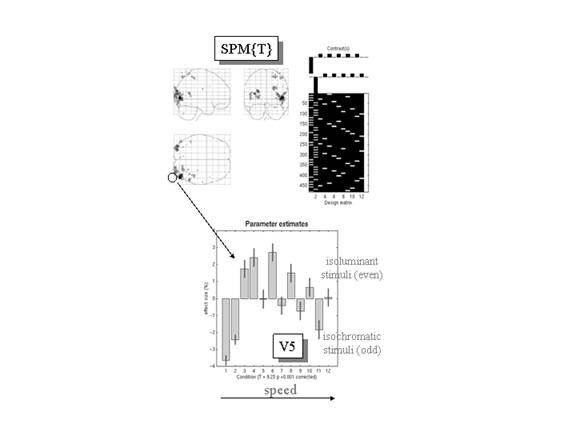Figure 8

Top right: Design matrix: This is an image representation of the design matrix. Contrasts: These are the vectors of contrast weights defining the linear compounds of parameters tested. The contrast weights are displayed over the column of the design matrix that corresponds to the effects in question. The design matrix here includes condition-specific effects (boxcars convolved with a hemodynamic response function). Odd columns correspond to stimuli shown under isochromatic conditions and even columns model responses to isoluminant stimuli. The first two columns are for stationary stimuli and the remaining columns are for conditions of increasing speed. The final column is a constant term. Top left: SPM{T}: This is a maximum intensity projection of the SPM{T} conforming to the standard anatomical space of Talairach and Tournoux (1988). The T values here are the minimum T values from both contrasts, thresholded at p = 0.001 uncorrected. The most significant conjunction is seen in left V5. Lower panel: Plot of the condition-specific parameter estimates for this voxel. The T value was 9.25 (p<0.001 corrected - see Figure 7.htm).